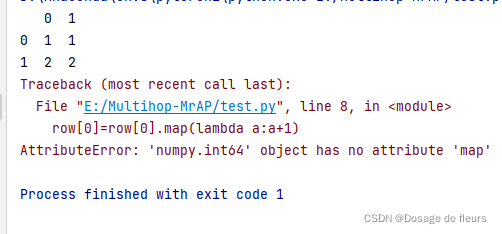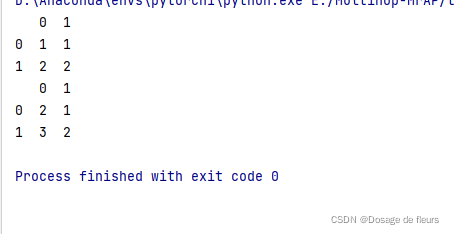# [Solved] AttributeError: ‘DataFrame‘ object has no attribute ‘map‘

AttributeError: ‘XXX’ object has no attribute ‘map’

Objects that meet the map format need to be in the form of key value pairs, so check whether the elements using the map function meet this condition.

For example:

``````#Create an object of DataFrame
list=[[1,1],[2,2]]
list=pd.DataFrame(list)
print(list)

#Use map to map them
for _, row in list.iterrows():
row=row.map(lambda a:a+1)
``````

Output:correct method:

``````list=[[1,1],[2,2]]
list=pd.DataFrame(list)
print(list)

list=list.map(lambda a:a+1)
print(list)
``````

Output: